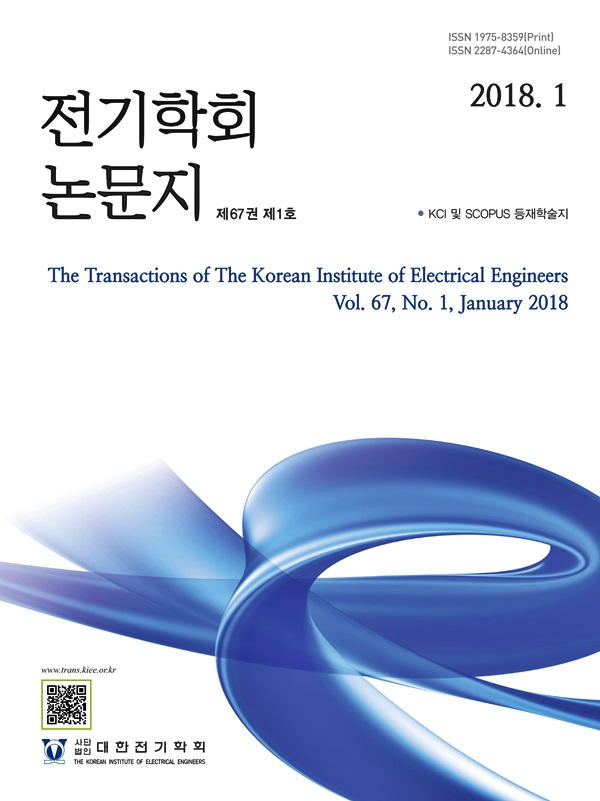Mobile QR Code#### The Transactions of the Korean Institute of Electrical Engineers

##### ISO Journal TitleTrans. Korean. Inst. Elect. Eng.
Title A Study on Short-circuit Capacity Calculation Method of Low-voltage Direct Current System Considering Short-circuit Characteristics of DC/DC Converter 오찬혁(Chan-Hyeok Oh) ; 이기연(Ki?Yeon Lee) ; 채동주(Dong?Ju Chae) ; 임승택(Seung?Taek Lim) https://doi.org/10.5370/KIEE.2023.72.11.1491 pp.1491-1497 1975-8359 Low-Voltage Direct Current; Short-Circuit Capacity; DC/DC Converter; Circuit Breaker In recent years, many studies have been conducted on DC distribution systems and commercialization attempts have been made, but they suffer from the lack of requirements for low-voltage direct current. This paper proposes a short-circuit capacity calculation method for low-voltage direct current systems by considering the short-circuit characteristics of DC/DC converters. In order to consider the different short-circuit fault behaviors of each converter, the equivalent capacitor capacity of the output terminal of the converter and the maximum output current of the converter are provided by the converter manufacturer. Using this, the short-circuit capacity is calculated by mixing the short-circuit characteristics of the converter output terminal capacitor and the short-circuit current supply characteristics due to the switching control of the converter. The proposed method is verified using Matlab/Simulink simulation to examine the appropriateness of the short-circuit capacity calculation method using the proposed method.